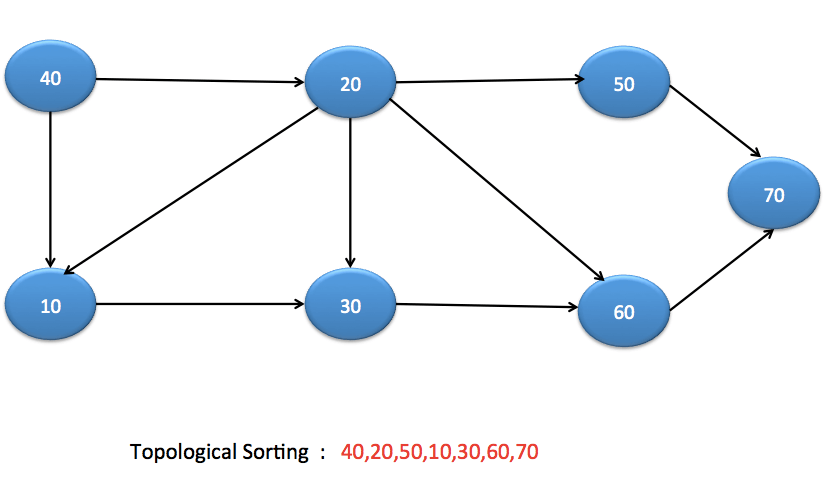# Topological Sort in java

In this post, we will see about Topological Sorting in the graph.

Topological Sorting is ordering of vertices or nodes such if there is an edge between (u,v) then u should come before v in topological sorting. Topological sort is possible only for Directed Acyclic Graph(DAG). If there is a cycle in graph, then there won’t be any possibility for Topological Sort.

## Topological Sort example

Let’s understand with the help of an example.
You might have used maven as build tool. If you have multiple modules in the maven, maven build projects on the basis of dependencies.
Let’s say you have 4 maven modules.

• maven-model
• mavan-util
• maven-ui

Now you need to build maven-model before maven-business-logic because maven-business-logic uses code from maven-model.
Similiarly, maven-model ,maven-business-logic and maven-util should be built before maven-ui as maven-ui depends on all three modules.

So, in this scenario, we can compute Topological sorting , so that maven can build them in the correct order.

## Topological Sort AlgorithmPlease note that there can be more than one solution for topological sort.

Let’s pick up node 30 here.

• Node 30 depends on node 20 and node 10.
• Node 10 depends on node 20 and node 40.
• Node 20 depends on node 40.

Hence node 10, node 20 and node 40 should come before node 30 in topological sorting.

This algorithm is a variant of Depth-first search. In depth first search, we first print the vertex and then go to its neighbours but  in case of topological sort, we don’t print vertex immediately instead we push it to the Stack.

In topological sorting, we will have a temporary stack. We are not going to print the vertex immediately, we first recursively call topological sorting for all its neighbour vertices, then push it to a stack. We will print stack once we are done with recursive topolgical sorting.

### Why it works?

It works because when you push any node to stack, you have already pushed its neighbours(and their neighbours and so on),so node which does not have any dependency will be on the top of stack. In our case, we will have 40 on top of the stack.

## Java program to implement topological sorting

When you run above program, you will get below output:

Topological Sorting Order:
40 20 50 10 30 60 70

## Time Complexity

It is very much similar to Depth-first search with just an extra stack. So its time complexity is O(V+E)

.That’s all about Topological Sort in java.